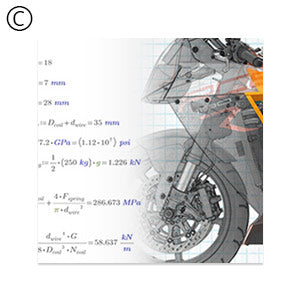0

| Authorized Reseller 16

# PTC Mathcad Worksheet Library - Applied Math

### 469 worksheets within five worksheet collections

Physical
Delivery

Available
in USA only

SKU: 13118

MPN: SPN-7579-L-

UPC:--The PTC Mathcad Worksheet Library - Applied Math consists of 469 worksheets within five worksheet collections. This worksheet library gives you ready-made PTC Mathcad templates that you can customize to perform a wide range of mathematical tasks, from solving equations to graphing and calculus.

## Worksheet Collections Content

Differential Equations (62 Worksheets)
The Differential Equations worksheets will provide the user with an overview of ordinary differential equations (ODEs). The worksheet collection is designed to show the user where ODEs come from and how to solve them in PTC Mathcad Prime 3.0. Topics covered include: boundary conditions, first and second order differential equations, homogeneous and nonhomogeneous differential equations, Euler's Method, Eigenvalues, linear and nonlinear differential equations, Bessel functions, and Legendre polynomials.
Statistics (77 Worksheets)
• Practical Statistics addresses the method of statistical decision making known as hypothesis testing as well as some of the methods involved in data analysis.
• The discussion of hypothesis testing in this collection serves two purposes:
1. To offer guidance in the setting up of hypothesis tests by taking a look at many of the common (and a few uncommon) tests used in practice.
2. To demonstrate, for both the occasional and professional statistician, how to perform hypothesis tests using Mathcad's functionality.
Within this collection, we will see how to:
• Select a random sample of data
• Form a frequency distribution
• Obtain descriptive measures
• Determine which type of analysis is appropriate
• Interpret our analysis results
Solving and Optimization (67 Worksheets)
To use PTC Mathcad's tools for solving and optimization problems, it is important to understand the mathematical methods behind them, the algorithms that implement these methods efficiently, and the limitations of performing these algorithms on a computer. The goal of this worksheet collection is to help you understand Mathcad's solving and optimization tools, and how to use them to create effective worksheets. Since different problems require different tools, this collection covers:
• Identifying the type of problem
• Choosing appropriate Mathcad functions for the problem
• Using the appropriate Mathcad functions correctly
• Interpreting the solutions
Specific examples are given throughout the collection, showing how best to use Mathcad in representative problems.
Data Analysis (67 Worksheets)
Data Analysis is a complex field comprising many disciplines. Understanding the statistics of your data helps you choose appropriate approximation tools, understand the limits of fit parameters, and discover new ways of analyzing your data. Often, the choice of a method is trial and error; sometimes it is based on physics, and sometimes just on intuition.

The purpose of this collection is not to give definitive answers about what methods are best for your data. That would be impossible. However, we have provided a variety of algorithms, utilities, and exploratory tools to help you choose quickly and efficiently between different mathematical approaches.
QuickSheets (196 Worksheets)
• Give you ready-made PTC Mathcad templates that you can customize to perform a wide range of mathematical tasks, from solving equations to graphing and calculus.
• Show you how to use some of PTC Mathcad's special features, like programming.
Individual Worksheets
• Complex Numbers in PTC Mathcad
• Cubic Spline Interpolation
• Developing IDF Curves from Rainfall Data
• Differential Algebraic Equations in PTC Mathcad
• Eigenvalues and Eigenvectors in PTC Mathcad
• Ergodicity in a Harmonic Oscillator
• Example of Using Laplace Transforms to Solve an ODE
• Example of Using Symbolic Math to Solve an ODE
• Financial Risk Management in PTC Mathcad
• Fitting Hyperbolic Data
• Gaussian Probability Distribution in PTC Mathcad
• Gauss_Newton Nonlinear Regression
• Generating Polar Histograms in PTC Mathcad
• Logistic Map and Ideal Random Number Generator
• Modeling the ocean
• Performing a Weighted Fit in PTC Mathcad
• Planar Regression in PTC Mathcad
• Random Triangles in the Unit Square
• Random Walks in PTC Mathcad
• Seeded Iteration in PTC Mathcad
• Solving Coupled ODEs in PTC Mathcad
• The Weibull Distribution Function in Reliability Statistics
• Tracking the Development of a Storm
• Monte Carlo Simulation of the Streeter Phelps Equation
• Inventory Control Example in PTC Mathcad
• Manufacturing Performance Curves in PTC Mathcad
• Plotting Frequency Distribution Data in PTC Mathcad
• Preparing Time Series for Data Analysis
• Using Cubic Splines to Determine Characteristics and Efficiency of a Pump

## Installation Requirements

In order to work properly, your copy of PTC Mathcad Worksheet Library Applied Math requires:PTC

No reviews yet
0%
(0)
0%
(0)
0%
(0)
0%
(0)
0%
(0)